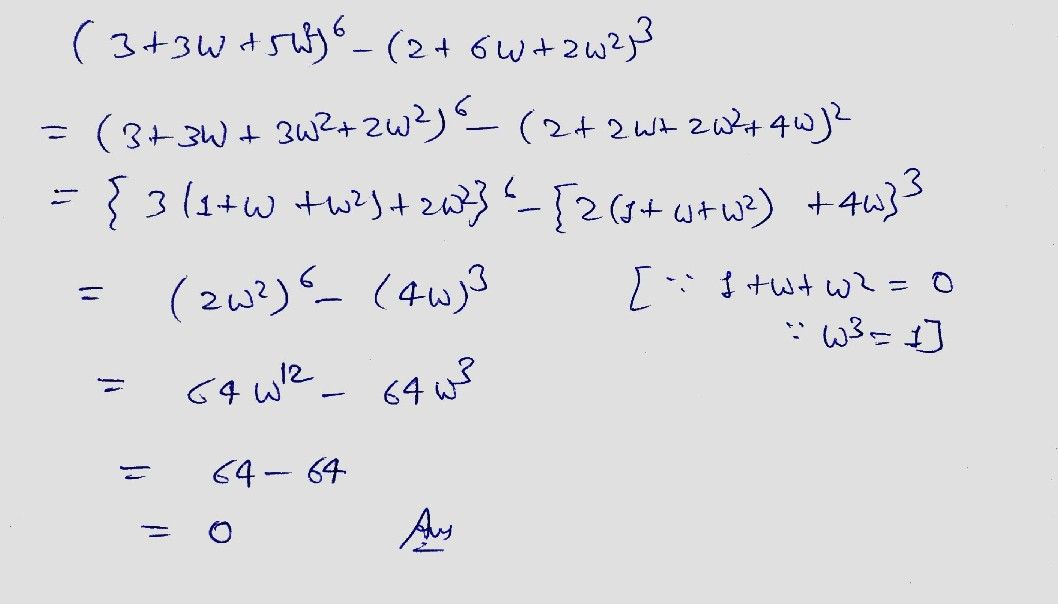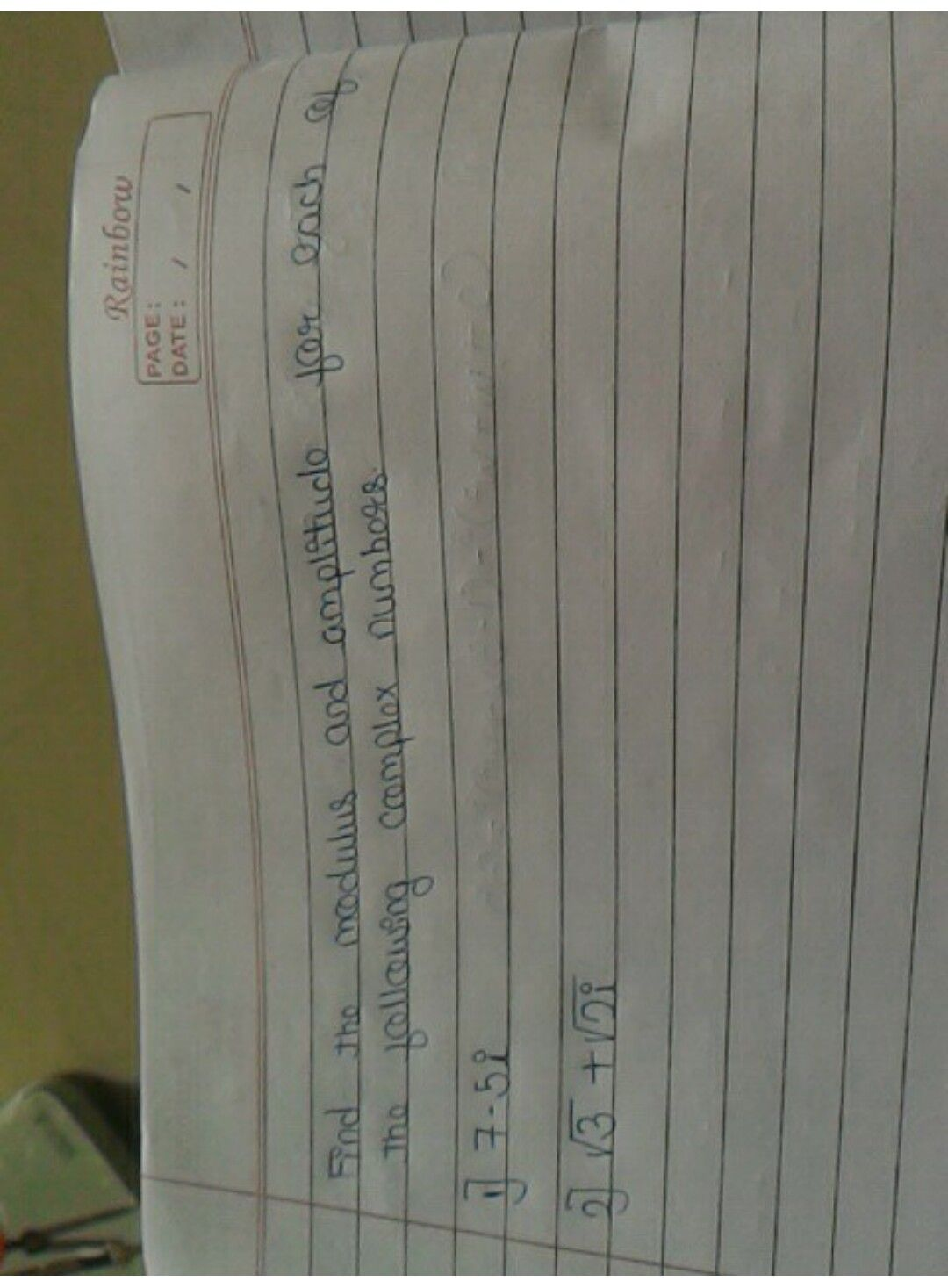Symbol
Problemshow. $\bar{I}$ IE380 Camplox aubo Kocats $4$ $xss^{°}x0$ $+c$ $9$ $\left(9+3$ $+s$ $2\right)8$ $\left(+6$ $+2$ $2\right)^{3}=0$ $2$ $\left(3+3$ $+5$ $2\right)^{6}-\left(2+6c$ $+n$ $2\right)^{3}=0$
Trigonometry
SolutionIf you have any doubt feel free to ask:) Dear, Please evaluate the answer and give gift coins(it does not reduce your coin balance.) for giving gift coins Click on evaluate. Then press the + icon 3 times(3000).
Please evaluate:- 1.Click on top right corner evaluate button. 2.Select 5 star 3.Click on gift coins + three times.(C3000)(not reduce your coins) 4. Click on DONE button on top right corner.Student